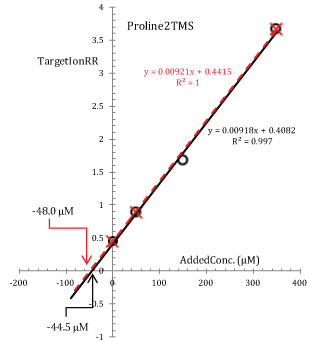Figure 4: Modified standard additions plot for Proline2TMS (target ion 142.2 Th; data from the main basic experiment in Figure 1). Experimental points are interpolated with a least square regression line whose equation is given in the plot (solid line). The broken line is the regression line calculated by excluding the experimental point at an added concentration of 150 μM.